$$\gdef\badDispatch#1{\textbf{\textcolor{e1432d}{#1}}} \gdef\noKatexForm#1{\badDispatch{#1}} \gdef\largeDot{\raisebox{0.06em}{\tiny∙}} \gdef\rarrbar{ {\raisebox{-0.05em}{→}\mkern{-0.13em}{\large\shortmid}}} \gdef\larrbar{ { {\large\shortmid}\mkern{-0.13em}{\raisebox{-0.05em}{←}}}} \gdef\suptrans{^\mathsf{T}} \gdef\supdagger{^\dagger} \gdef\rawsymbol#1{\operatorname{#1}} \gdef\colorsymbol#1#2{\textcolor{#1}{\rawsymbol{#2}}} \gdef\lsymbol#1{\colorsymbol{262626}{#1}} \gdef\msymbol#1{\colorsymbol{b50800}{#1}} \gdef\osymbol#1{\colorsymbol{00427f}{#1}} \gdef\lstring#1{\textcolor{666666}{\textrm{\textquotedblleft{\text{#1}}\textquotedblright}}} \gdef\boldForm#1{\textbf{#1}} \gdef\lrform#1{ {\textcolor{e1432d}{#1}}} \gdef\lgform#1{ {\textcolor{4ea82a}{#1}}} \gdef\lbform#1{ {\textcolor{3e81c3}{#1}}} \gdef\rform#1{ {\textcolor{cb2b1a}{#1}}} \gdef\gform#1{ {\textcolor{399318}{#1}}} \gdef\bform#1{ {\textcolor{2b6fb0}{#1}}} \gdef\drform#1{ {\textcolor{9b0000}{#1}}} \gdef\dgform#1{ {\textcolor{006800}{#1}}} \gdef\dbform#1{ {\textcolor{004987}{#1}}} \gdef\drgform#1{ {\textcolor{934100}{#1}}} \gdef\drgform#1{ {\textcolor{ae5900}{#1}}} \gdef\dgbform#1{ {\textcolor{006567}{#1}}} \gdef\drbform#1{ {\textcolor{86004d}{#1}}} \gdef\rgform#1{ {\textcolor{dc841a}{#1}}} \gdef\gbform#1{ {\textcolor{47a5a7}{#1}}} \gdef\rbform#1{ {\textcolor{c74883}{#1}}} \gdef\pform#1{ {\textcolor{665996}{#1}}} \gdef\dpform#1{ {\textcolor{41326b}{#1}}} \gdef\waform#1{ {\textcolor{6b6b6b}{#1}}} \gdef\wbform#1{ {\textcolor{929292}{#1}}} \gdef\wcform#1{ {\textcolor{c5c5c5}{#1}}} \gdef\tick{\checkmark} \gdef\barToken{\mathbf{|}} \gdef\filledRectangleToken{▮} \gdef\emptyRectangleToken{▯} \gdef\filledSquareToken{■} \gdef\emptySquareToken{□} \gdef\filledToken{∙} \gdef\emptyToken{∘} \gdef\cardinalRewrite#1#2{\rewrite{#1}{#2}} \gdef\primed#1{ {#1}^{\prime}} \gdef\tinybullet{ {\tiny●}} \gdef\forwardFactor{\uparrow} \gdef\backwardFactor{\downarrow} \gdef\neutralFactor{\mid} \gdef\arrowhead{⏵} \gdef\upArrowhead{⏶} \gdef\deSymbol{ { { {\raisebox{1.1pt}{\tinybullet}\mkern{-1pt}{→}}}\,}} \gdef\ueSymbol{ {\raisebox{1pt}{\tinybullet\raisebox{-0.03em}{\mkern{-0.3pt}{\tiny──}\mkern{-0.3pt}}\tinybullet}\,}} \gdef\ldeSymbol{ { { {\raisebox{1.1pt}{\tinybullet}\mkern{-1pt}{\longrightarrow}}}\,}} \gdef\lueSymbol{ {\raisebox{1pt}{\tinybullet\raisebox{-0.03em}{\mkern{-0.3pt}{\tiny────}\mkern{-0.3pt}}\tinybullet}\,}} \gdef\de#1#2{ { {#1} \deSymbol {#2}}} \gdef\ue#1#2{ { {#1} \ueSymbol {#2}}} \gdef\tde#1#2#3{ { {#1} \overset{#3}{\deSymbol} {#2}}} \gdef\tue#1#2#3{ { {#1} \overset{#3}{\ueSymbol} {#2}}} \gdef\ltde#1#2#3{ { {#1} \overset{#3}{\ldeSymbol} {#2}}} \gdef\ltue#1#2#3{ { {#1} \overset{#3}{\lueSymbol} {#2}}} \gdef\mapsfrom{\htmlClass{hreflect}{\mapsto}} \gdef\longmapsfrom{\htmlClass{hreflect}{\longmapsto}} \gdef\diffd{𝕕} \gdef\partialdof#1{\partial {#1}} \gdef\emptySet{\empty} \gdef\unknown{\wbform{\text{?}}} \gdef\notApplicable{\wbform{\text{---}}} \gdef\textAnd{\text{\,and\,}} \gdef\identicallyEqualSymbol{\equiv} \gdef\congruentSymbol{\equiv} \gdef\isomorphicSymbol{\simeq} \gdef\homeomorphicSymbol{\cong} \gdef\homotopicSymbol{\simeq} \gdef\approxEqualSymbol{\approx} \gdef\bijectiveSymbol{\approx} \gdef\defeq{\mathrel{≝}} \gdef\defEqualSymbol{\mathrel{≝}} \gdef\syntaxEqualSymbol{\mathrel{\textcolor{888888}{\equiv}}} \gdef\tailEqualSymbol{\underdot{=}} \gdef\headEqualSymbol{\dot{=}} \gdef\ruledelayed{:\to} \gdef\mathdollar{\text{\textdollar}} \gdef\hyphen{\text{-}} \gdef\endash{\text{--}} \gdef\emdash{\text{---}} \gdef\updownarrows{\uparrow\!\downarrow} \gdef\vthinspace{\mkern{1pt}} \gdef\dlq{\text{\textquotedblleft}} \gdef\drq{\text{\textquotedblright}} \gdef\dprime{ {\prime\prime}} \gdef\inverse#1{ { {#1}^{-1}}} \gdef\inverseSymbol{\inverse{□}} \gdef\groupDirectProduct#1{#1} \gdef\groupDirectProductSymbol{\times} \gdef\groupInverse{\inverse} \gdef\groupPower#1#2{ {#1}^{#2}} \gdef\groupCommutator#1#2{\left[{#1},{#2}\right]} \gdef\groupoidInverse{\inverse} \gdef\latticeBFS#1{\textrm{bfs}({#1})} \gdef\pathHomomorphism#1{#1} \gdef\groupoidFunction#1{#1} \gdef\groupoidHomomorphism#1{#1} \gdef\affineModifier#1{\overrightharpoon{#1}} \gdef\supercirc#1{#1^{\circ}} \gdef\supercircb#1{#1^{\bullet}} \gdef\blackCircleModifier#1{\supercircb{#1}} \gdef\whiteCircleModifier#1{\supercirc{#1}} \gdef\totalSpaceStyle#1{\rbform{#1}} \gdef\baseSpaceStyle#1{\bform{#1}} \gdef\fiberSpaceStyle#1{\rform{#1}} \gdef\totalSpaceElementStyle#1{\drbform{#1}} \gdef\baseSpaceElementStyle#1{\dbform{#1}} \gdef\fiberSpaceElementStyle#1{\drform{#1}} \gdef\bundleProjectionStyle#1{\dgform{#1}} \gdef\bundleGraphStyle#1{\rbform{#1}} \gdef\bundleSectionStyle#1{\drgform{#1}} \gdef\bundleFunctionStyle#1{\drbform{#1}} \gdef\graphFunctionStyle#1{\totalSpaceStyle{#1}} \gdef\projectionFunctionStyle#1{\totalSpaceStyle{#1}} \gdef\sectionFunctionStyle#1{\totalSpaceStyle{#1}} \gdef\functionGraph#1{G_{#1}} \gdef\toroidalModifier#1{\supercirc{#1}} \gdef\modulo#1{\supercirc{#1}} \gdef\dividesSymbol{\mathrel{|}} \gdef\groupFunction#1{#1} \gdef\groupHomomorphism#1{#1} \gdef\automorphisms{\operatorname{Aut}} \gdef\endomorphisms{\operatorname{End}} \gdef\transportMap#1{\transportMapSymbol_{#1}} \gdef\transportMapSymbol{\tau} \gdef\action#1{#1} \gdef\selfAction#1{\hat{#1}} \gdef\actionGroupoid#1{\utilde{#1}} \gdef\cardinalGroup#1{G^*({#1})} \gdef\signed#1{ {#1}^*} \gdef\transportAtlas#1{T_{#1}} \gdef\inverted#1{\underline{#1}} \gdef\mirror#1{\overline{#1}} \gdef\pathComposeSymbol{\mathbin{∷}} \gdef\pathCompose#1#2{ {#1}\pathComposeSymbol{#2}} \gdef\translateSymbol{\mathbin{\uparrow}} \gdef\backwardTranslateSymbol{\mathbin{\downarrow}} \gdef\pathTranslate#1#2{ {#1}\translateSymbol{#2}} \gdef\pathLeftTranslate#1{ { {#1}\translateSymbol}} \gdef\pathBackwardTranslate#1#2{ {#1}{\backwardTranslateSymbol}{#2}} \gdef\pathLeftBackwardTranslate#1{ {#1}{\backwardTranslateSymbol}} \gdef\compactCovariantDifference#1#2{\Delta_{#1}\,{#2}} \gdef\covariantDifference{ {#1}\,\Delta\,{#2}} \gdef\forwardDifference{\Delta^{+}} \gdef\backwardDifference{\Delta^{-}} \gdef\centralDifference{\Delta} \gdef\pathForwardDifference#1{\forwardDifference_{#1}} \gdef\pathBackwardDifference#1{\backwardDifference_{#1}} \gdef\pathCentralDifference#1{\centralDifference_{#1}} \gdef\pathHead#1{\pathHeadVector{#1}} \gdef\pathTail#1{\pathTailVector{#1}} \gdef\pathHeadVector#1{ {#1}^{\bullet}} \gdef\pathTailVector#1{ {#1}_{\bullet}} \gdef\pathReverse#1{ {#1}^{\dagger}} \gdef\pathIntegral#1#2{ {#1} \int {#2}} \gdef\pathIntegralSymbol{ {\int}} \gdef\pathDot#1#2{ {#1} \cdot {#2}} \gdef\pathDotSymbol{ {\cdot}} \gdef\compactBasis#1{\mathscr{B}} \gdef\length{\operatorname{len}} \gdef\signedLength{\operatorname{len^*}} \gdef\andFn{\operatorname{and}} \gdef\orFn{\operatorname{or}} \gdef\notFn{\operatorname{not}} \gdef\vertexList{\operatorname{vertices}} \gdef\vertexList{\operatorname{vertices}} \gdef\edgeList{\operatorname{edges}} \gdef\pathList{\operatorname{paths}} \gdef\cardinalList{\operatorname{cards}} \gdef\signedCardinalList{\operatorname{cards^*}} \gdef\wordOf{\operatorname{word}} \gdef\headVertex{\operatorname{head}} \gdef\tailVertex{\operatorname{tail}} \gdef\basis{\operatorname{basis}} \gdef\split{\operatorname{split}} \gdef\lcm{\operatorname{lcm}} \gdef\minimalContractionSets{\operatorname{MCSets}} \gdef\minimalContractions{\operatorname{MC}} \gdef\grade{\operatorname{grade}} \gdef\support{\operatorname{support}} \gdef\coefficient{\operatorname{coeff}} \gdef\domain{\operatorname{domain}} \gdef\codomain{\operatorname{codomain}} \gdef\modFunction{\operatorname{mod}} \gdef\clip{\operatorname{clip}} \gdef\sign{\operatorname{sign}} \gdef\step{\operatorname{step}} \gdef\projection{\operatorname{proj}} \gdef\lift{\operatorname{lift}} \gdef\identity{\operatorname{id}} \gdef\total{\operatorname{total}} \gdef\torus{\operatorname{torus}} \gdef\mobius{\operatorname{mobius}} \gdef\stateCompose{\operatorname{glue}} \gdef\infixStateComposeSymbol{\_} \gdef\stateDecompose{\operatorname{melt}} \gdef\stateJoin{\operatorname{conj}} \gdef\stateMeet{\operatorname{disj}} \gdef\stateExtent{\operatorname{extent}} \gdef\stateIntent{\operatorname{intent}} \gdef\infixStateJoinSymbol{\sqcup} \gdef\infixStateMeetSymbol{\sqcap} \gdef\isPrime#1{#1\textrm{ prime}} \gdef\blank{\_} \gdef\emptyWord{} \gdef\multiwordSymbol#1{\mathbf{#1}} \gdef\wordSymbol#1{\mathtt{#1}} \gdef\word#1{#1} \gdef\pathMap#1{#1} \gdef\function#1{#1} \gdef\imageModifier#1{ {#1}^{\rightarrow}} \gdef\preimageModifier#1{ {#1}^{\leftarrow}} \gdef\multiImageColorModifier#1{\msetCol{#1}} \gdef\multiImageModifier#1{ {#1}^{\Rightarrow}} \gdef\multiPreimageModifier#1{ {#1}^{\Leftarrow}} \gdef\functionComposition#1{#1} \gdef\functionCompositionSymbol{\mathbin{\small ∘}} \gdef\route#1#2#3{[{#1}\!:\!{#2}\!:\!{#3}]} \gdef\multiroute#1#2#3{[{#1}\!:\!{#2}\!:\!{#3}]} \gdef\pathWord#1#2#3{ {#1}\!:\!{#2}\!:\!{#3}} \gdef\nullPath{\bot} \gdef\nullElement{\bot} \gdef\path#1{#1} \gdef\vert#1{#1} \gdef\underdot#1{\underset{\raisebox{0.3em}{.}}{#1}} \gdef\tvert#1{\underdot{#1}} \gdef\hvert#1{\dot{#1}} \gdef\edge#1{#1} \gdef\card#1{\mathtt{#1}} \gdef\path#1{#1} \gdef\quiver#1{#1} \gdef\bindingRuleSymbol{\to} \gdef\compactBindingRuleSymbol{:} \gdef\cayleyQuiverSymbol#1{\selfAction{#1}} \gdef\bindCayleyQuiver#1#2{\selfAction{#1}[#2]} \gdef\bindActionQuiver#1#2{#1[#2]} \gdef\bindSize#1#2{#1(#2)} \gdef\bindCardSize#1#2{#1[#2]} \gdef\bindCards#1#2{#1[#2]} \gdef\subSize#1#2{#1_{#2}} \gdef\gridQuiver#1{\textrm{Grid}^{#1}} \gdef\treeQuiver#1{\textrm{Tree}^{#1}} \gdef\bouquetQuiver#1{\textrm{Bq}^{#1}} \gdef\lineQuiver{\textrm{L}} \gdef\cycleQuiver{\textrm{C}} \gdef\squareQuiver{\textrm{Sq}} \gdef\cubicQuiver{\textrm{Cbc}} \gdef\triangularQuiver{\textrm{Tri}} \gdef\hexagonalQuiver{\textrm{Hex}} \gdef\rhombilleQuiver{\textrm{Rmb}} \gdef\limit#1#2{\lim_{#2}\,#1} \gdef\realVectorSpace#1{\mathbb{R}^{#1}} \gdef\complexVectorSpace#1{\mathbb{C}^{#1}} \gdef\matrixRing#1#2{M_{#2}(#1)} \gdef\groupoid#1{#1} \gdef\group#1{#1} \gdef\field#1{#1} \gdef\ring#1{#1} \gdef\indeterminate#1{#1} \gdef\semiring#1{#1} \gdef\sym#1{#1} \gdef\matrix#1{#1} \gdef\polynomial#1{#1} \gdef\setLetter{\mathcal{S}} \gdef\signedSetLetter{\mathcal{S^*}} \gdef\multisetLetter{\mathcal{M}} \gdef\signedMultisetLetter{\mathcal{M^*}} \gdef\multisetSemiringSymbol#1{#1} \gdef\multisetSemiring#1#2{\multisetLetter\left[#1, #2\right]} \gdef\signedMultisetRingSymbol#1{#1} \gdef\signedMultisetRing#1#2{\signedMultisetLetter\left[#1, #2\right]} \gdef\polynomialRing#1#2{#1[{#2}]} \gdef\routeSymbol#1{#1} \gdef\multirouteSymbol#1{\mathbf{#1}} \gdef\planSymbol#1{\mathbf{#1}} \gdef\ringElement#1{#1} \gdef\matrixPart#1#2#3{#1\llbracket{#2,#3}\rrbracket} \gdef\matrixRowPart#1{#1} \gdef\matrixColumnPart#1{#1} \gdef\subMatrixPart#1#2#3{#1_{#2,#3}} \gdef\matrixDotSymbol{\cdot} \gdef\matrixPlusSymbol{+} \gdef\wordGroup#1{\wordGroupSymbol_{#1}} \gdef\wordGroupSymbol{\Omega} \gdef\wordRing#1{\wordRingSymbol_{#1}} \gdef\wordRingSymbol{\Omega\!\degree} \gdef\linearCombinationCoefficient#1{ {\textcolor{888888}{#1}}} \gdef\plan#1{#1} \gdef\planRing#1{\planRingSymbol_{#1}} \gdef\planRingSymbol{\Phi} \gdef\basisPath#1#2{\mathbf{#1}_{#2}} \gdef\basisPathWeight#1#2{ {#1}_{#2}} \gdef\unitSymbol{\mathbf{e}} \gdef\unitVertexField{\unitSymbol_1} \gdef\forwardSymbol{f} \gdef\backwardSymbol{b} \gdef\symmetricSymbol{s} \gdef\antisymmetricSymbol{a} \gdef\wordVector#1#2{\unitSymbol_{#1}^{#2}} \gdef\gradOf#1{\grad\,{#1}} \gdef\grad{\nabla} \gdef\divOf#1{\div\,{#1}} \gdef\div{\dot{\nabla}} \gdef\laplacianOf#1{\laplacian\,{#1}} \gdef\laplacian{\ddot{\nabla}} \gdef\suchThat#1#2{ {#1}\,\big|\,{#2}} \gdef\chart#1{\chartSymbol_{#1}} \gdef\chartSymbol{C} \gdef\graphRegionIntersectionSymbol{\cap} \gdef\graphRegionUnionSymbol{\cup} \gdef\pathIso{\simeq} \gdef\factorial#1{#1!} \gdef\power#1#2{ {#1}^{#2}} \gdef\repeatedPower#1#2{ {#1}^{#2}} \gdef\contractionLattice#1{\operatorname{Con}(#1)} \gdef\contractedRelation#1{\sim_{#1}} \gdef\isContracted#1#2{ {#1} \sim {#2}} \gdef\isContractedIn#1#2#3{ {#1} \sim_{#3} {#2}} \gdef\isNotContracted#1#2{ {#1} \not \sim {#2}} \gdef\isNotContractedIn#1#2#3{ {#1} \not \sim_{#3} {#2}} \gdef\contractionSum#1{#1} \gdef\contractionSumSymbol{\sqcup} \gdef\contractionProduct#1{#1} \gdef\contractionProductSymbol{ {\cdot}} \gdef\graph#1{#1} \gdef\graphHomomorphism#1{#1} \gdef\coversSymbol{\sqsupseteq} \gdef\coveredBySymbol{\sqsubseteq} \gdef\strictlyCoversSymbol{\sqsupset} \gdef\strictlyCoveredBySymbol{\sqsubset} \gdef\covering#1#2#3{ {#2} \sqsupseteq_{#1} {#3}} \gdef\powerSetSymbol{\mathcal{P}} \gdef\powerSet#1{\powerSetSymbol({#1})} \gdef\existsForm#1#2{\exists\,{#1}\,:\,{#2}} \gdef\forAllForm#1#2{\forall\,{#1}\,:\,{#2}} \gdef\notted#1{\notSymbol {#1}} \gdef\andSymbol{\land} \gdef\orSymbol{\lor} \gdef\notSymbol{\lnot} \gdef\latticeSymbol#1{#1} \gdef\meetSemilatticeSymbol#1{#1} \gdef\joinSemilatticeSymbol#1{#1} \gdef\posetSymbol#1{#1} \gdef\latticeElementSymbol#1{#1} \gdef\meetSemilatticeElementSymbol#1{#1} \gdef\joinSemilatticeElementSymbol#1{#1} \gdef\posetElementSymbol#1{#1} \gdef\latticeMeetSymbol{\wedge} \gdef\latticeJoinSymbol{\vee} \gdef\latticeTop{\top} \gdef\latticeBottom{\bot} \gdef\semilatticeMeetSymbol{\wedge} \gdef\semilatticeJoinSymbol{\vee} \gdef\semilatticeTop{\top} \gdef\semilatticeBottom{\bot} \gdef\semilatticeSemimeetSymbol{\wedge} \gdef\semilatticeSemijoinSymbol{\vee} \gdef\latticeGreaterSymbol{>} \gdef\latticeGreaterEqualSymbol{\ge} \gdef\latticeLessSymbol{<} \gdef\latticeLessEqualSymbol{\le} \gdef\posetGreaterSymbol{>} \gdef\posetGreaterEqualSymbol{\ge} \gdef\posetLessSymbol{<} \gdef\posetLessEqualSymbol{\le} \gdef\posetCoversSymbol{⋗} \gdef\posetCoveredBySymbol{⋖} \gdef\grpname#1{\operatorname{\mathsf{#1}}} \gdef\mkg#1#2#3{\grpname{#1}({#2},{#3})} \gdef\mka#1#2#3{\mathfrak{#1}({#2},{#3})} \gdef\generalLinearAlgebra#1#2{\mka{gl}{#1}{#2}} \gdef\generalLinearGroup#1#2{\mkg{GL}{#1}{#2}} \gdef\specialLinearAlgebra#1#2{\mka{sl}{#1}{#2}} \gdef\specialLinearGroup#1#2{\mkg{SL}{#1}{#2}} \gdef\projectiveGeneralLinearAlgebra#1#2{\mka{pgl}{#1}{#2}} \gdef\projectiveGeneralLinearGroup#1#2{\mkg{PGL}{#1}{#2}} \gdef\projectiveSpecialLinearAlgebra#1#2{\mka{psl}{#1}{#2}} \gdef\projectiveSpecialLinearGroup#1#2{\mkg{PSL}{#1}{#2}} \gdef\orthogonalAlgebra#1#2{\mka{o}{#1}{#2}} \gdef\orthogonalGroup#1#2{\mkg{O}{#1}{#2}} \gdef\specialOrthogonalAlgebra#1#2{\mka{so}{#1}{#2}} \gdef\specialOrthogonalGroup#1#2{\mkg{SO}{#1}{#2}} \gdef\unitaryAlgebra#1{\mka{u}{#1}{#2}} \gdef\unitaryGroup#1{\mkg{U}{#1}{#2}} \gdef\specialUnitaryAlgebra#1#2{\mka{su}{#1}{#2}} \gdef\specialUnitaryGroup#1#2{\mkg{su}{#1}{#2}} \gdef\spinAlgebra#1#2{\mka{spin}{#1}{#2}} \gdef\spinGroup#1#2{\mkg{Spin}{#1}{#2}} \gdef\pinAlgebra#1#2{\mka{pin}{#1}{#2}} \gdef\pinGroup#1#2{\mkg{Pin}{#1}{#2}} \gdef\symmetricGroup#1{\grpname{Sym}({#1})} \gdef\presentation#1{#1} \gdef\groupPresentation#1#2{\left\langle\,{#1}\,\,\middle|\mathstrut\,\,{#2}\,\right\rangle} \gdef\groupRelationIso{=} \gdef\groupGenerator#1{#1} \gdef\groupRelator#1{#1} \gdef\groupElement#1{#1} \gdef\identityElement#1{#1} \gdef\groupoidElement#1{#1} \gdef\groupIdentity#1{#1} \gdef\groupoidIdentity#1{#1} \gdef\ringIdentity#1{#1} \gdef\iconstruct#1#2{ {#1}\,\,\middle|{\large\mathstrut}\,\,{#2}} \gdef\setConstructor#1#2{\left\{\,\iconstruct{#1}{#2}\,\right\}} \gdef\multisetConstructor#1#2{\left\{\mkern{-2.3pt}\left|\,\,\iconstruct{#1}{#2}\,\right|\mkern{-2.3pt}\right\}} \gdef\cardinalityConstructor#1#2{\left|\,\iconstruct{#1}{#2}\,\right|} \gdef\setToMultiset#1{ {#1}^\uparrow} \gdef\multisetToSet#1{ {#1}^\downarrow} \gdef\subsets#1{\setLetter({#1})} \gdef\signedSubsets#1{\signedSetLetter({#1})} \gdef\multisets#1{\multisetLetter({#1})} \gdef\signedMultisets#1{\signedMultisetLetter({#1})} \gdef\circleSpaceSymbol{S} \gdef\topologicalSpace#1{#1} \gdef\bundleSection#1{#1} \gdef\bundleProjection#1{#1} \gdef\setSymbol#1{#1} \gdef\signedSetSymbol#1{#1} \gdef\multisetSymbol#1{#1} \gdef\signedMultisetSymbol#1{#1} \gdef\setElementSymbol#1{#1} \gdef\signedSetElementSymbol#1{#1} \gdef\multisetElementSymbol#1{#1} \gdef\signedMultisetElementSymbol#1{#1} \gdef\negated#1{\bar{#1}} \gdef\positiveSignedPart#1{ {#1}^+} \gdef\negativeSignedPart#1{ {#1}^-} \gdef\multisetMultiplicitySymbol{\,\raisebox{.1em}{\small\#}\mkern{.1em}\,} \gdef\signedMultisetMultiplicitySymbol{\,\raisebox{.1em}{\small\#}\mkern{.1em}\,} \gdef\boundMultiplicityFunction#1{ {#1}^{\sharp}} \gdef\boundSignedMultiplicityFunction#1{\operatorname{ {#1}^{\sharp}}} \gdef\constructor#1#2{\left.{#1}\,\,\middle|\mathstrut\,\,{#2}\right.} \gdef\elemOf#1#2{ { {#1} \in {#2} }} \gdef\notElemOf#1#2{ { {#1} \notin {#2} }} \gdef\edgeOf#1#2{ { {#1} {\in}_E {#2} }} \gdef\vertOf#1#2{ { {#1} {\in}_V {#2} }} \gdef\pathOf#1#2{ { {#1} {\in}_P {#2} }} \gdef\pathType#1{\operatorName{path}{#1}} \gdef\vertexType#1{\operatorName{vertex}{#1}} \gdef\edgeType#1{\operatorName{edge}{#1}} \gdef\multisetType#1{\operatorName{mset}{#1}} \gdef\signedMultisetType#1{\operatorName{mset^*}{#1}} \gdef\unitInterval{\mathbb{I}} \gdef\fromTo#1{#1} \gdef\fromToSymbol{\mapsto} \gdef\vertexCountOf#1{|{#1}|} \gdef\vertices#1{ V_{#1} } \gdef\edges#1{ E_{#1} } \gdef\vertexField#1{#1} \gdef\edgeField#1{#1} \gdef\pathVector#1{\mathbf{#1}} \gdef\pathVectorSpace#1{\mathscr{#1}} \gdef\baseField#1{#1} \gdef\finiteField#1{\mathbb{F}_{#1}} \gdef\functionSpace#1#2{#2^{#1}} \gdef\finiteTotalFunctionSpace#1#2{#2^{\sub #1}} \gdef\pathGroupoid#1{ { \Gamma_{#1} }} \gdef\forwardPathQuiver#1#2{ {\overrightharpoon{#1}_{#2}}} \gdef\backwardPathQuiver#1#2{ {\overrightharpoon{#1}^{#2}}} \gdef\pathQuiver#1{ {\overrightharpoon{#1}}} \gdef\mto#1#2{ {#1}\mapsto{#2}} \gdef\mtoSymbol{\mapsto} \gdef\groupWordRewriting#1{\langle{#1}\rangle} \gdef\rewrite#1#2{ {#1}\mapsto{#2}} \gdef\rewritingRule#1#2{ {#1}\mapsto{#2}} \gdef\rewritingSystem#1{\mathcal{#1}} \gdef\multiwayBFS#1{\textrm{bfs}({#1})} \gdef\rewritingStateBinding#1#2{ {\left.{#1}\!\mid\!{#2}\right.}} \gdef\rewritingRuleBinding#1#2{#1{\left[\,{#2}\,\right]}} \gdef\namedSystem#1{\mathtt{#1}} \gdef\genericRewritingSystem{\namedSystem{Sys}} \gdef\stringRewritingSystem{\namedSystem{Str}} \gdef\circularStringRewritingSystem{\namedSystem{CStr}} \gdef\turingMachineRewritingSystem{\namedSystem{TM}} \gdef\cellularAutomatonRewritingSystem{\namedSystem{CA}} \gdef\graphRewritingSystem{\namedSystem{Gr}} \gdef\hypergraphRewritingSystem{\namedSystem{HGr}} \gdef\petriNetRewritingSystem{\namedSystem{Petri}} \gdef\localStates#1{\localStatesSymbol^#1} \gdef\regionalStates#1{\regionalStatesSymbol^#1} \gdef\globalStates#1{\globalStatesSymbol^#1} \gdef\keySubStates#1{\keySubStatesSymbol^#1} \gdef\valueSubStates#1{\valueSubStatesSymbol^#1} \gdef\localState#1#2{#2_{#1}} \gdef\regionalState#1{#1} \gdef\globalState#1{#1} \gdef\keySubState#1{#1} \gdef\valueSubState#1{#1} \gdef\lhsState#1{#1_{L}} \gdef\rhsState#1{#1_{R}} \gdef\rewriteLHSRegionalState#1{#1_{L}} \gdef\rewriteRHSRegionalState#1{#1_{R}} \gdef\regionalStateForm#1{\waform{(}#1\waform{)}} \gdef\invalidRegionalState{\rform{\times}} \gdef\emptyRegionalState{\regionalStateForm{}} \gdef\regionalSubstateSymbol{\sqsubseteq} \gdef\regionalSuperstateSymbol{\sqsupseteq} \gdef\comparableRegionalStatesSymbol{\mathbin{\square}} \gdef\incomparableRegionalStatesSymbol{\mathbin{\boxtimes}} \gdef\namedStateSet#1{\mathbf #1} \gdef\localStatesSymbol{\namedStateSet L} \gdef\regionalStatesSymbol{\namedStateSet R} \gdef\globalStatesSymbol{\namedStateSet G} \gdef\keySubStatesSymbol{\namedStateSet L_K} \gdef\valueSubStatesSymbol{\namedStateSet L_V} \gdef\localStateSymbol#1{#1} \gdef\regionalStateSymbol#1{#1} \gdef\globalStateSymbol#1{#1} \gdef\keySubStateSymbol#1{#1} \gdef\valueSubStateSymbol#1{#1} \gdef\infixComposeLocalStatesSymbol{\_} \gdef\composeLocalStatesSymbol{\operatorname{glue}} \gdef\composeLocalStatesForm#1{\composeLocalStatesSymbol(#1)} \gdef\ncard#1{\card{\inverted{#1}}} \gdef\mcard#1{\card{\mirror{#1}}} \gdef\nmcard#1{\card{\inverted{\mirror{#1}}}} \gdef\assocArray#1{\left\langle {#1} \right\rangle} \gdef\openMultiset{\lBrace} \gdef\closeMultiset{\rBrace} \gdef\set#1{\{ {#1} \}} \gdef\signedSet#1{\{ {#1} \}} \gdef\list#1{\{ {#1} \}} \gdef\multiset#1{\openMultiset {#1} \closeMultiset} \gdef\signedMultiset#1{\openMultiset {#1} \closeMultiset} \gdef\styledSet#1#2{#1\{ {#1} #1\}} \gdef\styledList#1#2{#1\{ {#1} #1\}} \gdef\styledMultiset#1#2{#1\openMultiset {#2} #1\closeMultiset} \gdef\styledSignedMultiset#1#2{\openMultiset {#2} \closeMultiset} \gdef\permutationCycle#1{#1} \gdef\permutationCycleSymbol{\to} \gdef\permutationSet#1{#1} \gdef\permutationSetSymbol{;} \gdef\transposition#1#2{ {#1} \leftrightarrow {#2}} \gdef\tuple#1{( {#1} )} \gdef\concat#1{ {#1} } \gdef\paren#1{\left( {#1} \right)} \gdef\ceiling#1{\lceil{#1}\rceil} \gdef\floor#1{\lfloor{#1}\rfloor} \gdef\translationVector#1{\left[{#1}\right]_{\textrm{T}}} \gdef\quotient#1#2{ {#1} / {#2}} \gdef\compactQuotient#1#2#3{ {#1}\;{\upharpoonright_{#2}}\;{#3}} \gdef\multilineQuotient#1#2{\frac{#1}{#2}} \gdef\idElem#1{e_{#1}} \gdef\minus#1{-{#1}} \gdef\elem{\ \in\ } \gdef\vsp{\mkern{0.4pt}} \gdef\iGmult{\vsp} \gdef\gmult{\vsp\ast\vsp} \gdef\Gmult{\vsp\ast\vsp} \gdef\gdot{\vsp\cdot\vsp} \gdef\gDot{\vsp\mathbin{\largeDot}\vsp} \gdef\mdot{\vsp\cdot\vsp} \gdef\smallblackcirc{\vsp\raisebox{0.15em}{\tiny∙}\vsp} \gdef\smallwhitecirc{\vsp\raisebox{0.15em}{\tiny∘}\vsp} \gdef\sgdot{\mathbin{\smallwhitecirc}} \gdef\srdot{\mathbin{\smallblackcirc}} \gdef\srplus{+} \gdef\ellipsis{\,\wbform{...}\,} \gdef\verticalEllipsis{\vdots} \gdef\appliedRelation#1{#1} \gdef\setUnionSymbol{\cup} \gdef\setIntersectionSymbol{\cap} \gdef\setRelativeComplementSymbol{-} \gdef\msetCol{\textcolor{bb4444}} \gdef\repeatedMultiset#1#2{#1\,#2} \gdef\msrdot{\mathbin{\smallblackcirc}} \gdef\msrplus{+} \gdef\smrdot{\mathbin{\smallblackcirc}} \gdef\smrplus{+} \gdef\dotminus{\mathbin{\dot{-}}} \gdef\dotcap{\mathbin{\dot{\cap}}} \gdef\dotcup{\mathbin{\dot{\cup}}} \gdef\multisetUnionSymbol{\dotcup} \gdef\multisetIntersectionSymbol{\dotcap} \gdef\multisetRelativeComplementSymbol{\dotminus} \gdef\multisetSumSymbol{\dotplus} \gdef\cartesianProductSymbol{\times} \gdef\functionType#1#2{ {#1} \to {#2}} \gdef\functionSignature#1#2#3{ { {#1} : {#2} \to {#3}}} \gdef\partialFunctionSignature#1#2#3{ { {#1} : {#2} \rightharpoonup {#3}}} \gdef\poly#1{#1} \gdef\quiverProdPoly#1{#1} \gdef\quiverProdPower#1#2{#1^{#2}} \gdef\quiverProdTimes#1{#1} \gdef\parenLabeled#1#2{#1\ ({#2})} \gdef\parenRepeated#1#2{\parenLabeled{#1}{ {#2}\text{ times}}} \gdef\underLabeled#1#2{\underbrace{#1}_{#2}} \gdef\underRepeated#1#2{\underbrace{#1}_{#2\text{ times}}} \gdef\overLabeled#1#2{\overbrace{#1}^{#2}} \gdef\overRepeated#1#2{\overbrace{#1}^{#2\text{ times}}} \gdef\modLabeled#1#2{ {#1 }\textrm{ mod }{#2}} \gdef\freeGroup#1{F_{#1}} \gdef\cyclicGroup#1{\mathbb{Z}_{#1}} \gdef\componentSuperQuiverOfSymbol{\succ} \gdef\setCardinality#1{|{#1}|} \gdef\multisetCardinality#1{|{#1}|} \gdef\dependentQuiverProductSymbol{\mathbin{\times}} \gdef\independentQuiverProductSymbol{\mathbin{⨝}} \gdef\graphUnionSymbol{\sqcup} \gdef\graphProductSymbol{\times} \gdef\inlineProdSymbol{|} \gdef\serialCardSymbol{ {:}} \gdef\parallelCardSymbol{ {\mid}} \gdef\cardinalSequenceSymbol{ {:}} \gdef\cardinalProductSymbol{\inlineProdSymbol} \gdef\vertexProductSymbol{\inlineProdSymbol} \gdef\edgeProductSymbol{\inlineProdSymbol} \gdef\indexSum#1#2#3{ {\sum_{#2}^{#3} #1}} \gdef\indexProd#1#2#3{ {\prod_{#2}^{#3} #1}} \gdef\indexMax#1#2#3{ {\max_{#2}^{#3} #1}} \gdef\indexMin#1#2#3{ {\min_{#2}^{#3} #1}} \gdef\indexUnion#1#2#3{ {\bigcup_{#2}^{#3} #1}} \gdef\indexIntersection#1#2#3{ {\bigcap_{#2}^{#3} #1}} \gdef\styledIndexSum#1#2#3#4{ {#1\sum_{#3}^{#4} #2}} \gdef\styledIndexProd#1#2#3#4{ {#1\prod_{#3}^{#4} #2}} \gdef\styledIndexMax#1#2#3#4{ {#1\max_{#3}^{#4} #2}} \gdef\styledIndexMin#1#2#3#4{ {#1\min_{#3}^{#4} #2}} \gdef\indexSumSymbol{\sum} \gdef\indexProdSymbol{\prod} \gdef\indexMaxSymbol{\max} \gdef\indexMinSymbol{\min} \gdef\openInterval#1#2{(#1,#2)} \gdef\closedInterval#1#2{[#1,#2]} \gdef\openClosedInterval#1#2{(#1,#2]} \gdef\closedOpenInterval#1#2{[#1,#2)} \gdef\oneTo#1{1..{#1}} \gdef\zeroTo#1{0..{#1}} \gdef\qstring#1{\mathtt{"}{#1}\mathtt{"}} \gdef\wstring#1{\textcolor{6b6b6b}{#1}} \gdef\qchar#1{\mathtt{'}{#1}\mathtt{'}} \gdef\lstr#1{\mathtt{#1}} \gdef\lchar#1{\mathtt{#1}} \gdef\string#1{ {#1}} \gdef\character#1{ {#1}} \gdef\homomorphismMapping#1{\assocArray{#1}} \gdef\translationPresentation#1{\textrm{Z}_{#1}} \gdef\starTranslationPresentation#1{\textrm{Z}^*_{#1}} \gdef\translationPathValuation#1{\mathcal{\overrightharpoon Z}_{#1}} \gdef\starTranslationPathValuation#1{\overrightharpoon{\mathcal{Z}^*_{#1}}} \gdef\translationWordHomomorphism#1{\mathcal{Z}_{#1}} \gdef\starTranslationWordHomomorphism#1{\mathcal{Z}^*_{#1}} \gdef\translationCardinalValuation#1{\textrm{T}_{#1}} \gdef\starTranslationCardinalValuation#1{\textrm{T}^*_{#1}}$$
Contraction lattice

Contraction lattice

Recap

In the previous section Coverings, we introduced the idea of a quiver covering. Starting from a single quiver $$\quiver{Q}$$ we considered the contraction order of $$\quiver{Q}$$, whose elements are contracted quivers in which sets of vertices of $$\quiver{Q}$$ are identified with one another. In this section we will develop this idea further, turning the contraction order into a contraction lattice, and study the structure of this lattice for some of the basic transitive quivers. We will also outline the connections of this lattice with group theory.

Contraction sets and terms

Let's start with the quiver $$\bindCards{\subSize{\squareQuiver }{2}}{\rform{\card{r}},\bform{\card{b}}}$$, shown below: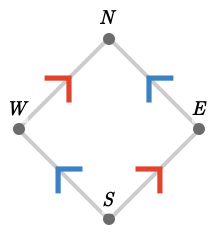The contraction order for this quiver is shown below, where each contracted quiver is labeled with the corresponding contraction set, which we will define shortly: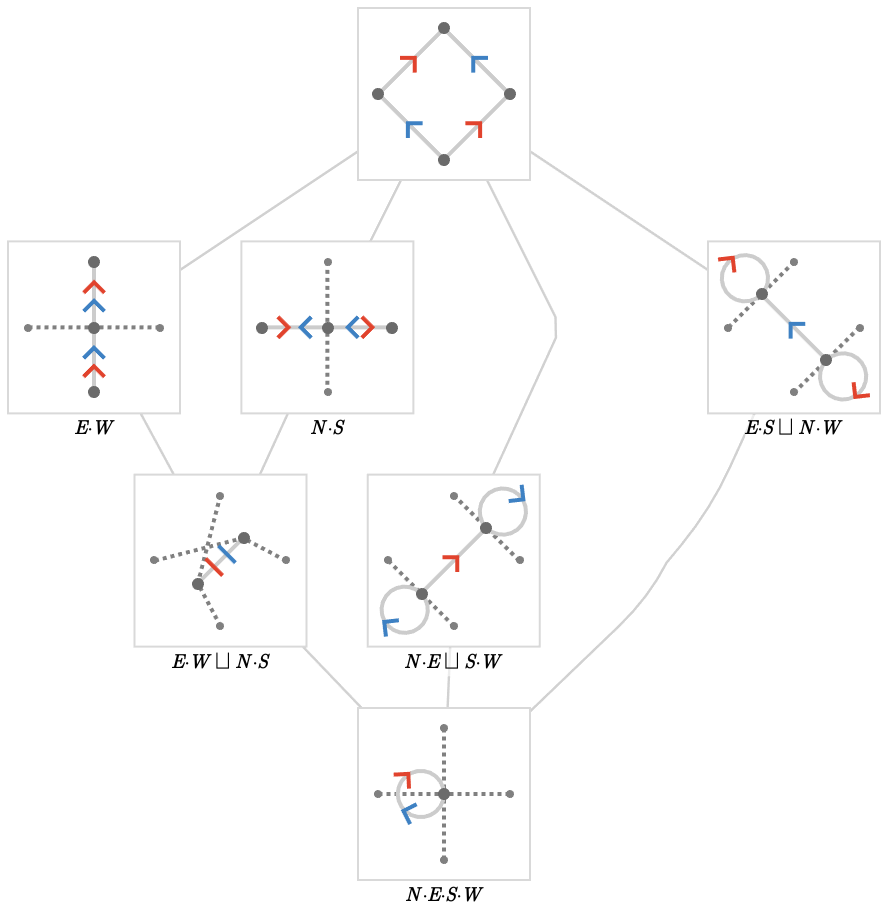A contraction set $$\sym{S}$$ expresses a contraction of the original quiver in terms of a disjoint union of contraction terms $$\sym{T}_{\sym{i}}$$:

$\sym{S} = \contractionSum{\sym{T}_1\contractionSumSymbol \sym{T}_2\contractionSumSymbol \ellipsis \contractionSumSymbol \sym{T}_{\sym{n}}}$

Each term $$\sym{T}_{\sym{i}}$$ represents a set of vertices that are contracted together. For example, the contracted quiver on the right is specified by the contraction set $$\contractionSum{\contractionProduct{\vert{E}\contractionProductSymbol \vert{S}}\contractionSumSymbol \contractionProduct{\vert{N}\contractionProductSymbol \vert{W}}}$$, which consists of the disjoint contraction terms $$\contractionProduct{\vert{E}\contractionProductSymbol \vert{S}}$$ and $$\contractionProduct{\vert{N}\contractionProductSymbol \vert{W}}$$.

Visualizing contractions

We'll introduce three ways to visualize the individual contracted quivers in the contraction lattice. To illustrate these visualizations, we'll use the same contractions of $$\bindCards{\subSize{\squareQuiver }{2}}{\rform{\card{r}},\bform{\card{b}}}$$ that we introduced above: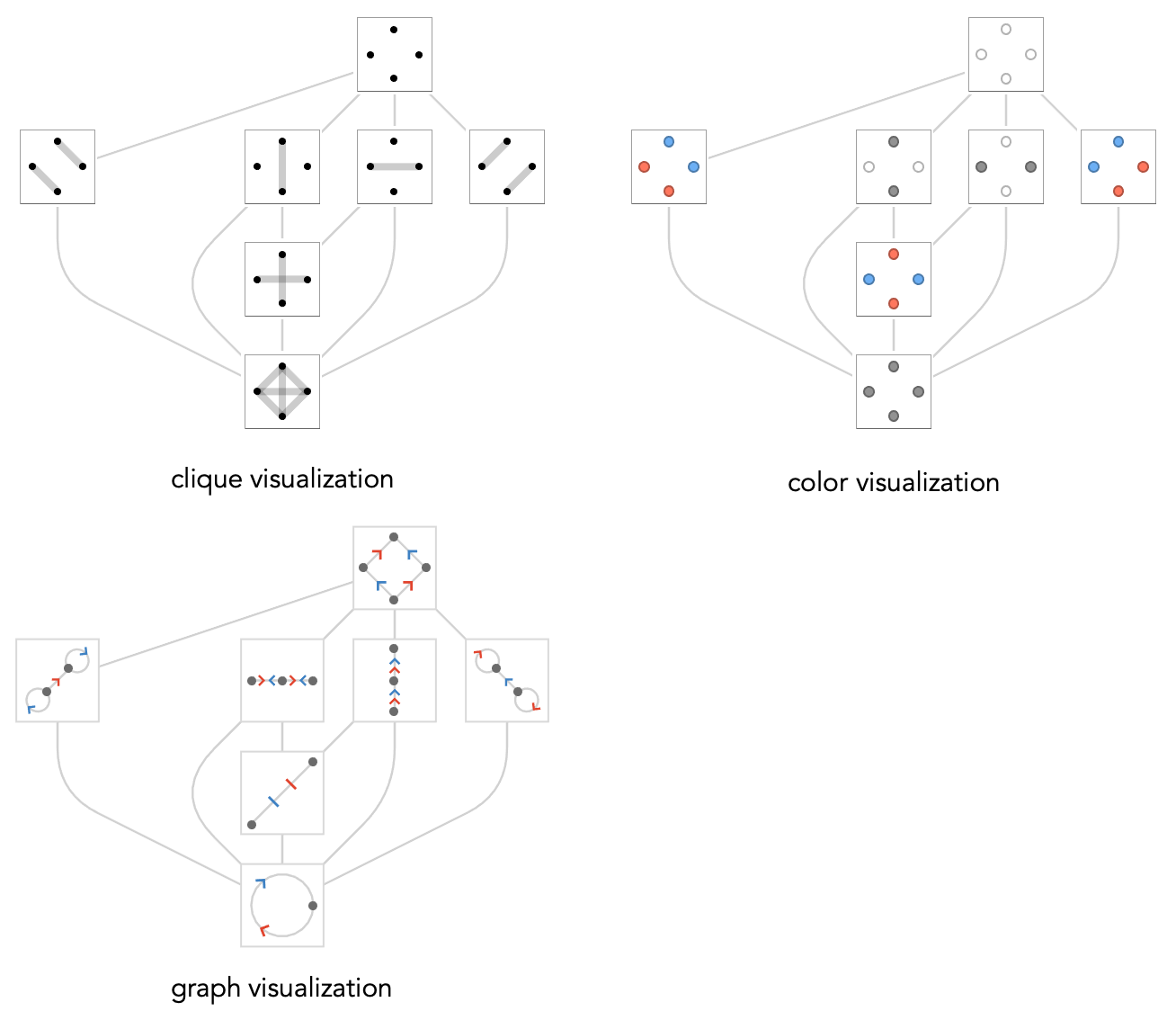The clique visualization depicts each contraction term as a clique of interconnected vertices. The color visualization draws vertices belonging to the same contraction term using distinct colors (note these colors have no connection to the colors of the cardinals), and uses white for vertices that are left uncontracted. The graph visualization shows the contracted graphs directly.

Lattice structure

We defined the contraction order to be the partially ordered set of contracted quivers of some original quiver $$\quiver{Q}$$. Next we will show how to to attach the structure of an order-theoretic lattice to this poset, which we'll call the contraction lattice of $$\quiver{Q}$$, written $$\contractionLattice{\quiver{Q}}$$.

Lattices

First we give a superficial summary of lattices, but we recommend reading additional material about lattices to make the most of this section.

A lattice is a poset with two additional binary operations, known as $$meet$$ $$\latticeMeetSymbol$$ and join $$\latticeJoinSymbol$$. These operations distribute over each other, and play a role analogous to intersection and union of sets – in particular the subsets of a set $$\sym{X}$$ form a lattice in which $$\latticeElement{A}\latticeMeetSymbol \latticeElement{B}\defEqualSymbol \latticeElement{A}\setIntersectionSymbol \latticeElement{B}$$, $$\latticeElement{A}\latticeJoinSymbol \latticeElement{B}\defEqualSymbol \latticeElement{A}\setUnionSymbol \latticeElement{B}$$, and $$\latticeElement{A} \le \latticeElement{B}\iff \latticeElement{A} \subseteq \latticeElement{B}$$. A bounded lattice is a lattice with two distinguished elements called top $$\latticeTop$$ and bottom $$\latticeBottom$$, which are respectively greater than and less than all other elements, and serve as the identity for meet and join respectively.

Contractions as equivalence relations

Recall that $$\path{R}$$ is a contraction quiver of $$\quiver{Q}$$ if the vertices of $$\path{R}$$ are contractions of vertices of $$\quiver{Q}$$ that preserve the local uniqueness property. We can then see $$\path{R}$$ as being uniquely specified by an equivalence relation on the vertices of $$\quiver{Q}$$. We’ll write this relation as $$\isContractedIn{\vert{u}}{\vert{v}}{\quiver{R}}$$, which is the statement that vertices $$\vert{u},\vert{v}$$ of $$\quiver{Q}$$ are considered contracted together in $$\quiver{R}$$.

A little thought will reveal that a contraction $$\quiver{R}$$ covers another contraction $$\quiver{S}$$, written $$\quiver{R}\coversSymbol \quiver{S}$$ (or in lattice terminology $$\quiver{R}\latticeGreaterEqualSymbol \quiver{S}$$) if and only if the relation for $$\quiver{R}$$ is a refinement of the relation for $$\quiver{S}$$:

$\quiver{R}\coversSymbol \quiver{S}\iff \paren{\isContractedIn{\vert{u}}{\vert{v}}{\quiver{S}}\implies \isContractedIn{\vert{u}}{\vert{v}}{\quiver{R}}}$

(Note that we use the word "cover" here in the usual sense of a quiver covering, not in the lattice-theoretic sense of a cover, which has a different meaning.)

Ok, we've expressed the elements of the contraction order in terms of equivalence relations on sets, with the partial order induced by refinement of equivalence relations. The finest equivalence relation regards all vertices as distinct, corresponding to the uncontraction $$\quiver{Q}$$, and the coarsest equivalence relation regards all vertices as contracted, corresponding to the complete contraction, given by a bouquet quiver with the same cardinals as $$\quiver{Q}$$. These serve as the top and bottom of the contraction order: $$\latticeTop = \quiver{Q},\latticeBottom = \bouquetQuiver{\sym{k}}$$.

Topped intersection structure

We now show that the set of vertex equivalence relations describing valid quiver contractions form a subset of the powerset of the vertices, closed under intersection. A set of this form is called a topped intersection structure, and automatically gives this set the structure of a bounded lattice. The meet and join are defined as you would expect: the meet of two relations corresponds to the finest relation that is still coarser than both, and the join of two relations gives the coarsest relation that still is finer than both.

First, we show that if we have two quiver contraction equivalence relations $$\contractedRelation{\quiver{R}},\contractedRelation{\quiver{S}}$$, the set-theoretic intersection of these two relations (as subsets of the powerset) $$\contractedRelation{\quiver{R} \, \quiver{S}}\defEqualSymbol \contractedRelation{\quiver{R}}\setIntersectionSymbol \contractedRelation{\quiver{S}}$$ is again an equivalence relation. This is straightforward:

\begin{aligned} \paren{\isContractedIn{\vert{u}}{\vert{v}}{\quiver{R} \, \quiver{S}}}\andSymbol \paren{\isContractedIn{\vert{v}}{\vert{w}}{\quiver{R} \, \quiver{S}}} & \implies \paren{\isContractedIn{\vert{u}}{\vert{v}}{\quiver{R}}}\andSymbol \paren{\isContractedIn{\vert{u}}{\vert{v}}{\quiver{R}}}\andSymbol \paren{\isContractedIn{\vert{v}}{\vert{w}}{\quiver{S}}}\andSymbol \paren{\isContractedIn{\vert{v}}{\vert{w}}{\quiver{S}}}\\ & \implies \paren{\isContractedIn{\vert{u}}{\vert{w}}{\quiver{R}}}\andSymbol \paren{\isContractedIn{\vert{u}}{\vert{w}}{\quiver{S}}}\\ & \implies \isContractedIn{\vert{u}}{\vert{w}}{\quiver{R} \, \quiver{S}}\\ \end{aligned}

It remains to be shown that this equivalence relation corresponds to a quiver contraction, meaning that it preserves LU. We use proof by contradiction: assume that we have a violation of LU witnessed by $$\tde{\vert{u}}{\vert{v}}{\card{c}},\tde{\vert{u}}{\vert{w}}{\card{c}},\isNotContractedIn{\vert{v}}{\vert{w}}{\quiver{R} \, \quiver{S}}$$, where $$\vert{u},\vert{v},\vert{w}$$ are arbitrary representatives of equivalence classes in the relation $$\contractedRelation{\quiver{R} \, \quiver{S}}$$. Since $$\contractedRelation{\quiver{R}}$$ and $$\contractedRelation{\quiver{S}}$$ both do correspond to quiver contractions, we must have both $$\isContractedIn{\vert{v}}{\vert{w}}{\quiver{R}}$$ and $$\isContractedIn{\vert{v}}{\vert{w}}{\quiver{S}}$$ to prevent these being witnesses to violation of LU in $$\quiver{R}$$ and $$\quiver{S}$$ respectively. But this implies that $$\isContractedIn{\vert{v}}{\vert{w}}{\quiver{R} \, \quiver{S}}$$ by definition, a contradiction.

Intuition

In terms of contractions, we can provide an intuitive summary of this situation as follows:

• the meet $$\quiver{R}\latticeMeetSymbol \quiver{S}$$ of two contracted quivers gives the contracted quiver that performs all the vertex contractions present in either, as well as the minimum number of additional contractions that is required to recover LU. In other words, the “minimal amount of contraction” that will unify both quivers.

• the join $$\quiver{R}\latticeJoinSymbol \quiver{S}$$ of two contracted quivers gives the contracted quiver that has undergone as much contraction as possible that is consistent with either of them: any additional contractions would contract pairs of vertices that are not contracted in one or the other.

Minimal contraction sets

We now describe a simple algorithm to find the minimal contraction sets of a quiver $$\quiver{Q}$$. These are “minimal” in the sense that they are forced by a contraction of only two vertices. We'll write this set as $$\minimalContractionSets(\quiver{Q})$$, and the corresponding contracted quivers as $$\minimalContractions(\quiver{Q})$$.

The algorithm starts by enumerating the set of all possible contaction terms containing two distinct vertices. Each 2-term serves as a "seed" for calculating a minimal contraction set that preserves local uniqueness (abbreviated LU). We first contract the vertices of the original quiver using the seed 2-term, and then test if this produces any duplicate incoming or outgoing cardinals, as these indicate a violation of LU for one or more cardinals. If there are no duplicates, we are done, and have a new minimal contraction set containing just that single 2-term, and we can move on to the next candidate term.

If not, we "chase" all duplicate incoming or outgoing cardinals, and so obtain one or more additional 2-terms that must be contracted to re-establish the LU. This chasing process might have to repeat several times, since these additional contraction terms will eliminate the original violations of LU but could introduce new violations for the new terms. When we finally obtain a new set of contraction terms that does not introduce any new LU violations, we are done, and we have a minimal contraction set, and can proceed to the next candidate pair.

Example

We'll use $$\bindSize{\squareQuiver }{2,3}$$ to illustrate this process: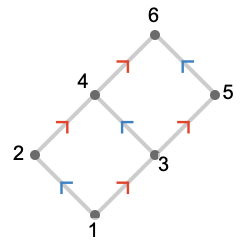Here we show a seed term $$\contractionProduct{1\contractionProductSymbol 2}$$ and the successive steps of the "growing" of the seed, each time chasing outgoing $$\rform{\card{r}}$$-duplicates: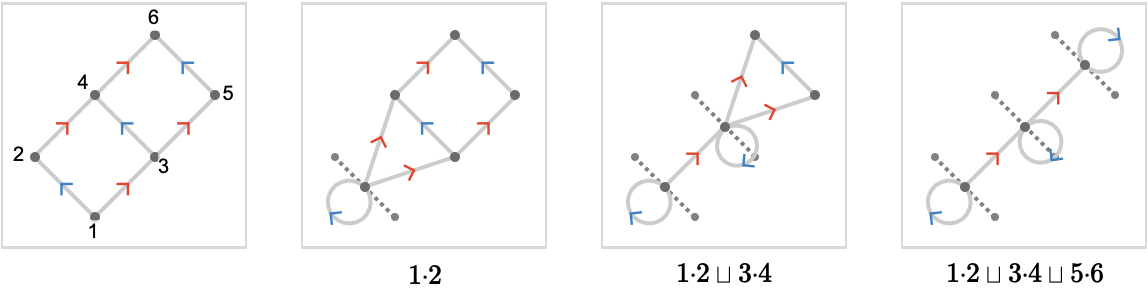Here we show the sequence for a different seed term $$\contractionProduct{1\contractionProductSymbol 3}$$: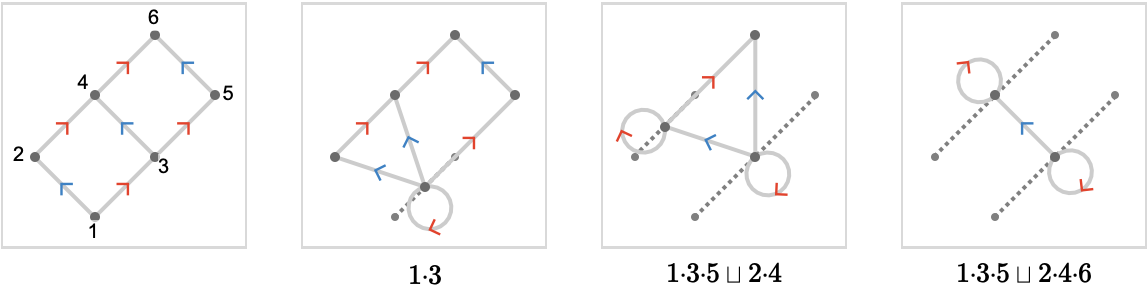The sequence for $$\contractionProduct{2\contractionProductSymbol 3}$$: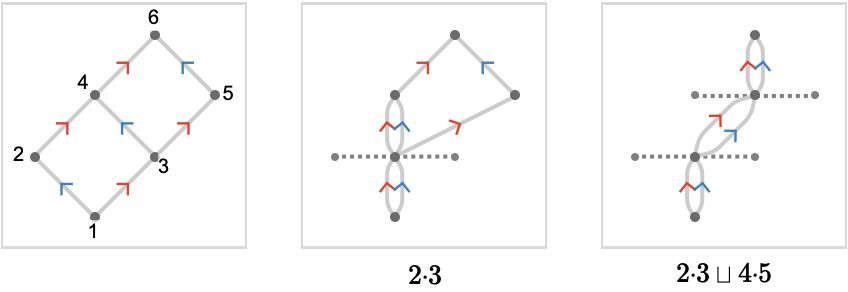Notice that if we start at the seed $$\contractionProduct{4\contractionProductSymbol 5}$$, we obtain the same final contraction: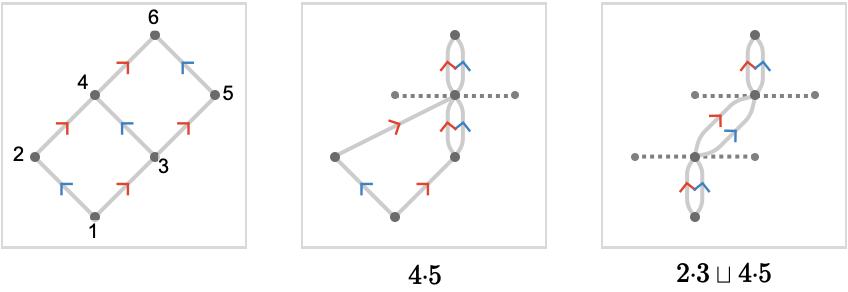With a little thought it becomes clear that we will grow the same contraction set when starting from any of its terms. This implies that our enumeration algorithm can avoid this redundancy by skipping any candidate 2-terms that have already appeared as a 2-term of any previously generated contraction set.

Some simple minimal contractions

To gain intuition, we'll explore the minimal contraction sets $$\minimalContractionSets(\quiver{Q})$$ for the line, cycle, square, and square tori quivers. These will turn out to be straightforward to understand, and in fact will give us a good initial understanding of the nature of the contraction lattices for these particular quivers.

Cycle quiver

Here we show $$\minimalContractionSets(\subSize{\cycleQuiver }{12})$$, using color visualization, and below each, the corresponding minimal contracted quivers $$\minimalContractions(\subSize{\cycleQuiver }{12})$$: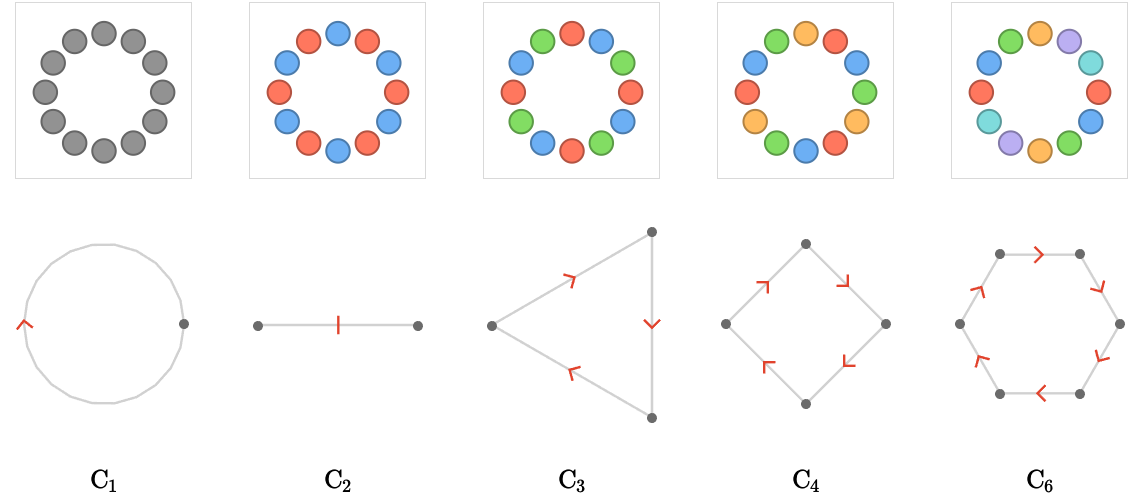We've also labeled each of the contracted quivers with its "closed form", that is, a named quiver that is isomoprhic to the contracted quiver. These are the cycle quivers whose size consists of prime power divisors of 12, plus the "degenerate prime" $$\subSize{\cycleQuiver }{1}$$. This turns out to be true for the minimal contraction sets of $$\subSize{\cycleQuiver }{\sym{n}}$$ for arbitrary $$\sym{n}$$, yielding the theorem:

$\minimalContractions(\subSize{\cycleQuiver }{\sym{n}}) = \setConstructor{\subSize{\cycleQuiver }{\power{\sym{p}}{\sym{m}}}}{\elemOf{\sym{m}}{\mathbb{N}^+},\elemOf{\sym{p}}{\mathbb{P}},\power{\sym{p}}{\sym{m}}\dividesSymbol \sym{n}}\setUnionSymbol \list{\subSize{\cycleQuiver }{1}}$

Here $$\mathbb{P}$$ stands for the domain of the prime numbers.

Line quiver

Here we show $$\minimalContractionSets(\subSize{\lineQuiver }{7})$$: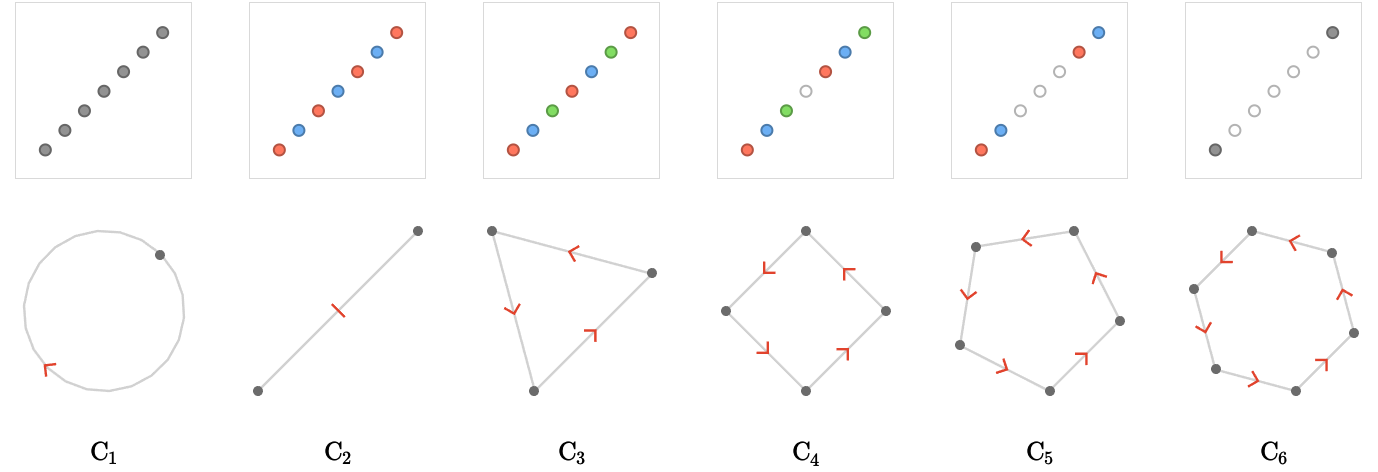The minimal contractions here the ways to glue the two ends of the line together with more or less overlap, yielding cycle quivers. Again, the pattern generalizes, yielding the following theorem:

$\minimalContractions(\subSize{\lineQuiver }{\sym{n}}) = \setConstructor{\subSize{\cycleQuiver }{\sym{i}}}{1 \le \sym{i}<\sym{n}}$

Square quiver

We'll start with $$\bindSize{\squareQuiver }{3,2}$$, the example we worked through earlier:Here is $$\minimalContractionSets(\bindSize{\squareQuiver }{3,2})$$: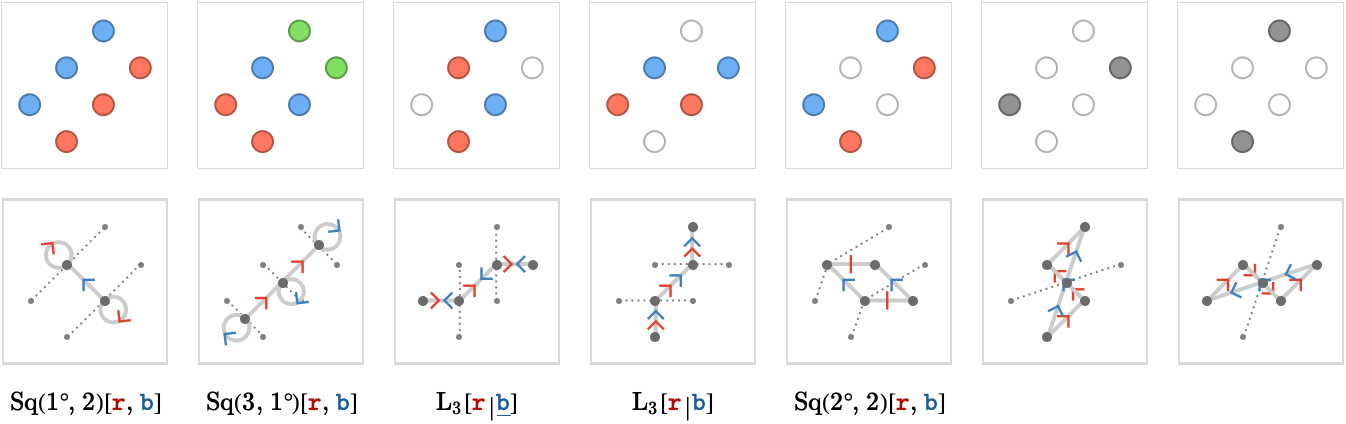Notice that the first 5 contractions have intuitive interpretations. The $$1^{\textrm{st}}$$, $$2^{\textrm{nd}}$$ and $$5^{\textrm{th}}$$ have "rolled up" the square quiver to form toroidal lattices in either the $$\rform{\card{r}}$$ or $$\bform{\card{b}}$$ axis. The $$3^{\textrm{rd}}$$ and $$4^{\textrm{th}}$$ have "projected" the 2D square lattice into a 1D lattice with the two possibily "parities". To explain the final two contractions, we will consider the larger example of $$\bindSize{\squareQuiver }{3,3}:$$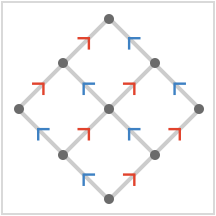The twelve generating contraction sets are shown below using color visualization: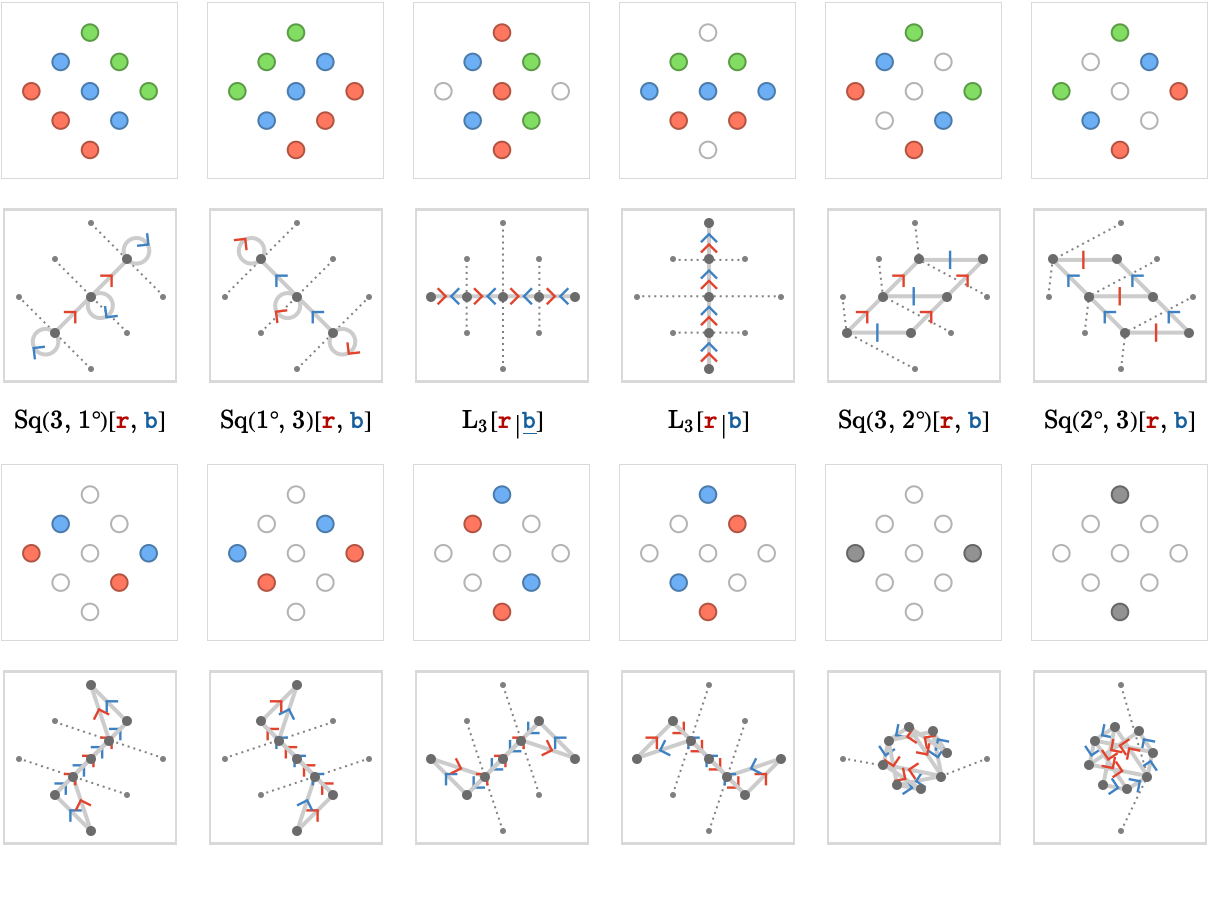As before, we obtain several torodial contractions and two linear contractions. A little thought will see that we can compose the remaining, mysterious contractions with each other to obtain sheared toroidal contractions with shear factors of $$1$$ and $$-1$$ along either the $$\rform{\card{r}}$$ or $$\bform{\card{b}}$$ axes.

Square tori

Earlier, we saw that a line quiver contracted to cycle quivers, which themselves contracted to other cycle quivers according to their prime power factors. Similarly, the square quiver contracted to line quivers and (possibly sheared) square tori, which are the 2D analogues of the cycle quivers. So do the square tori contract to other square tori?

To answer this question, we compute the minimal contractions for some small square tori: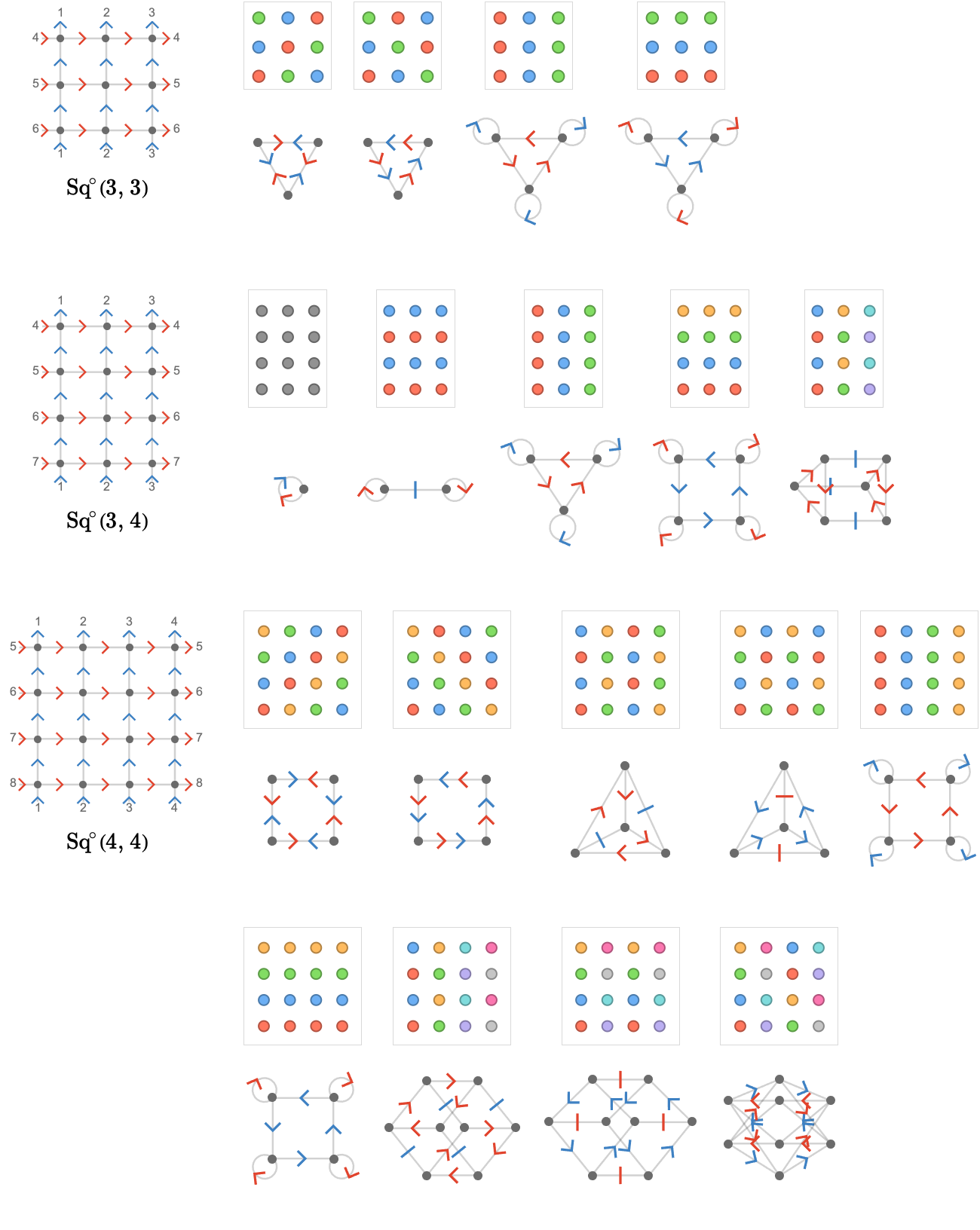The answer here is a provisional yes. Eliding cardinal assingments for brevity, we have the following closed forms, given in the same order as the above diagrams:

\begin{aligned} \minimalContractions(\bindSize{\toroidalModifier{\squareQuiver }}{3,3}) &= \list{\bindSize{\lineQuiver }{3},\bindSize{\lineQuiver }{3},\bindSize{\toroidalModifier{\squareQuiver }}{3,1},\bindSize{\toroidalModifier{\squareQuiver }}{1,3}}\\ \minimalContractions(\bindSize{\toroidalModifier{\squareQuiver }}{3,4}) &= \list{\bindSize{\cycleQuiver }{1},\bindSize{\toroidalModifier{\squareQuiver }}{1,2},\bindSize{\toroidalModifier{\squareQuiver }}{3,1},\bindSize{\toroidalModifier{\squareQuiver }}{2,4},\bindSize{\toroidalModifier{\squareQuiver }}{3,2}}\\ \minimalContractions(\bindSize{\toroidalModifier{\squareQuiver }}{4,4}) &= \list{\bindSize{\cycleQuiver }{4},\bindSize{\toroidalModifier{\squareQuiver }}{2_1,2},\bindSize{\toroidalModifier{\squareQuiver }}{2,2_1},\bindSize{\toroidalModifier{\squareQuiver }}{4,1},\bindSize{\toroidalModifier{\squareQuiver }}{1,4},\bindSize{\toroidalModifier{\squareQuiver }}{4,2},\bindSize{\toroidalModifier{\squareQuiver }}{2,4},\bindSize{\toroidalModifier{\squareQuiver }}{2_2,2}}\\ \end{aligned}

Obtaining the contraction lattice

Having obtained the set of minimal contraction sets for a quiver, how do we go about generating the entire contraction lattice?

To gain intuition for this problem, let's look at the lattice for the quiver $$\bindSize{\squareQuiver }{2,3}$$ we looked at initially. Here we highlight the vertices that correspond to the minimal contraction sets: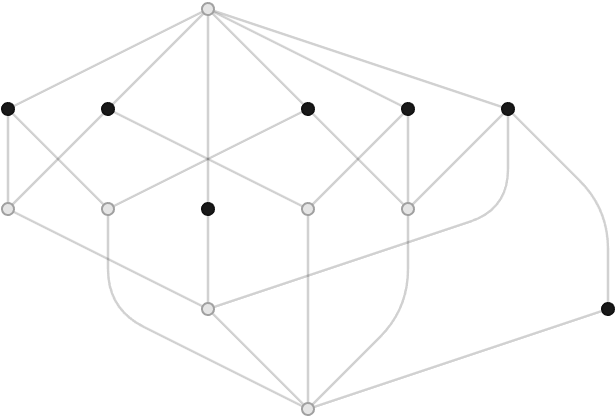The minimal contractions here seem to be precisely the elements that are covered by a single other element. Lattice elements with this property are known as meet-irreducible in order theory. Meet-irreducible elements have the further property that for complete lattices, every element can be expressed as the meet of meet-irreducible elements (although not necessarily in a unique way).

The non-uniqueness of this decomposition can be seen in the example above. The rightmost element on the third row can be formed by taking the meet of any 2 of its 3 parents (or all three). The situation can be even more complicated: the left element on the penultimate row has 4 meet-irreducible parents. There are 5 ways of taking the meet of 2 of these parents to form it, but there is also 1 way of taking the meet of 2 of its parents (the left 2) that does not form it. Taking the meet of any 3 of its parents is sufficient to form it, however.

Nevertheless, this connection with meet-irreducibility suggests a plain if inelegant way generate all other contraction sets: we can form all possible sums of the meet irreducible contraction sets. This corresponds to forming a valid contraction $$\quiver{R}\coveredBySymbol \quiver{Q}$$ as the meet of every minimal contraction generated by all pairs of contracted vertices in $$\quiver{R}$$.

Modular representation theory